# 一、概述

## 1.1 背景

• BI平台面向的用户及应用场景
• 如何使用BI平台进行数据的分析与展示
• BI取数的实现原理

## 1.2 面向用户及应用场景

BI平台面向的用户主要包括：

• 报表开发者：一般是BI分析师和数据开发同学
• 报表使用者：包括但不限于运营、服务、产品等任何对数据有可视化分析需求的同学
• 其他内部应用：有数据展示或数据分析需求的其他产品

# 二、可视化分析数据

## 2.1 术语简介

• 维度：数据观察的角度，一般为分类数据，如日期、店铺等。具体又可分为行维和列维，如图1.2所示
• 行维相当于excel表格中的表头列
• 列维相当于excel表格中数值列上方的多行表头
• 数值：又称指标，是多维数组的取值。是特定维度下要统计的指标的聚合所得到的的值，一般为数值类型的数据，如销售额、利润等。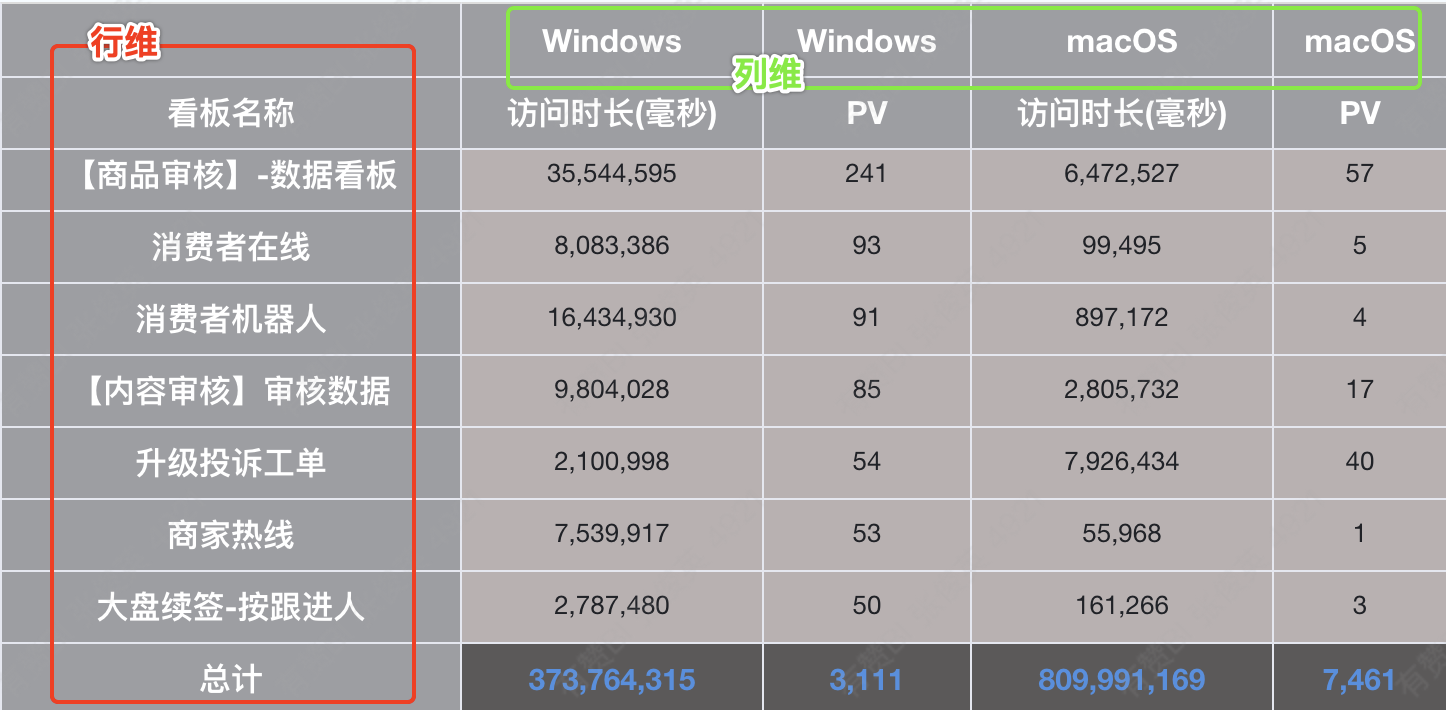图1.2 维度类型

## 2.2 快速入门### step1: 添加数据集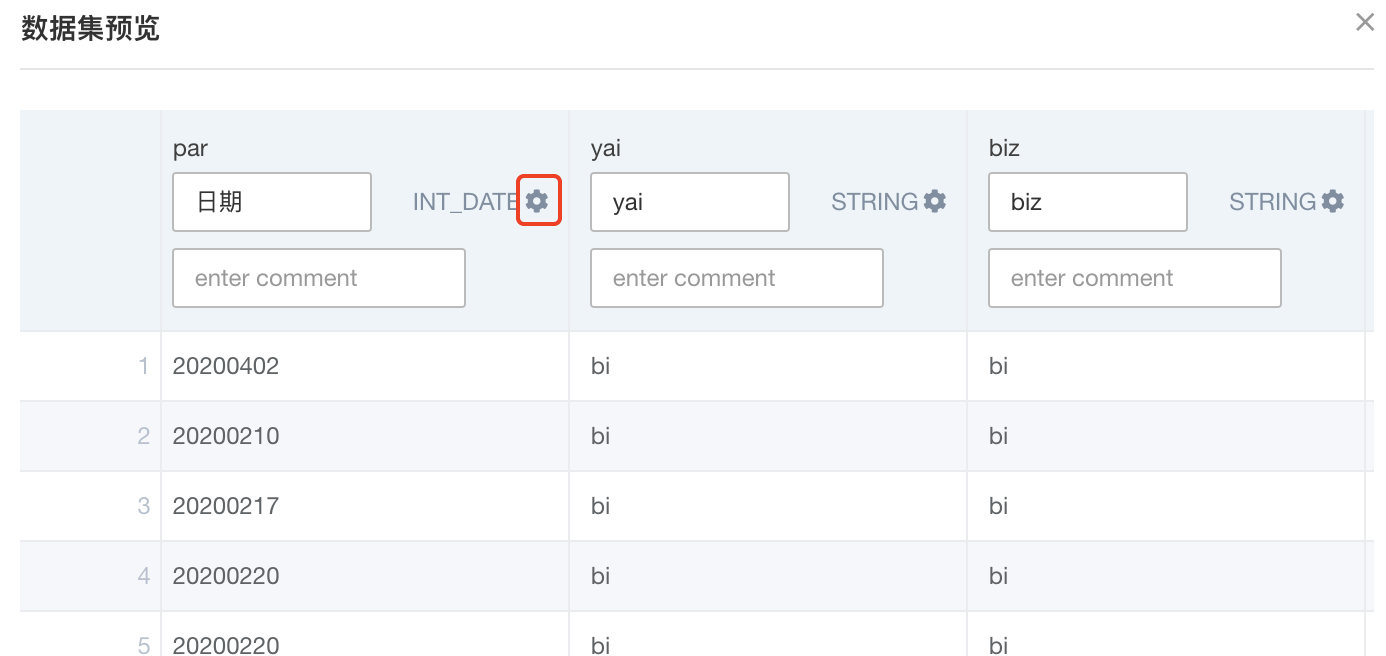小技巧
1.可在字段的右上角齿轮处标记字段的日期类型及格式，当标记为日期类型时，可使用日期的时间粒度，时间组件等。


### step2: 添加报表

小技巧
1.添加计算字段：适合需要二次加工计算的指标
如转化率 = count(XXX) / count(XXX)（相当于Excel pivot里的计算字段）
2.报表复制功能
场景：已有报表"top GMV的营销活动"， 需要添加 "top买家数的营销活动"，
此时便可使用报表的复制功能


### step3: 添加权限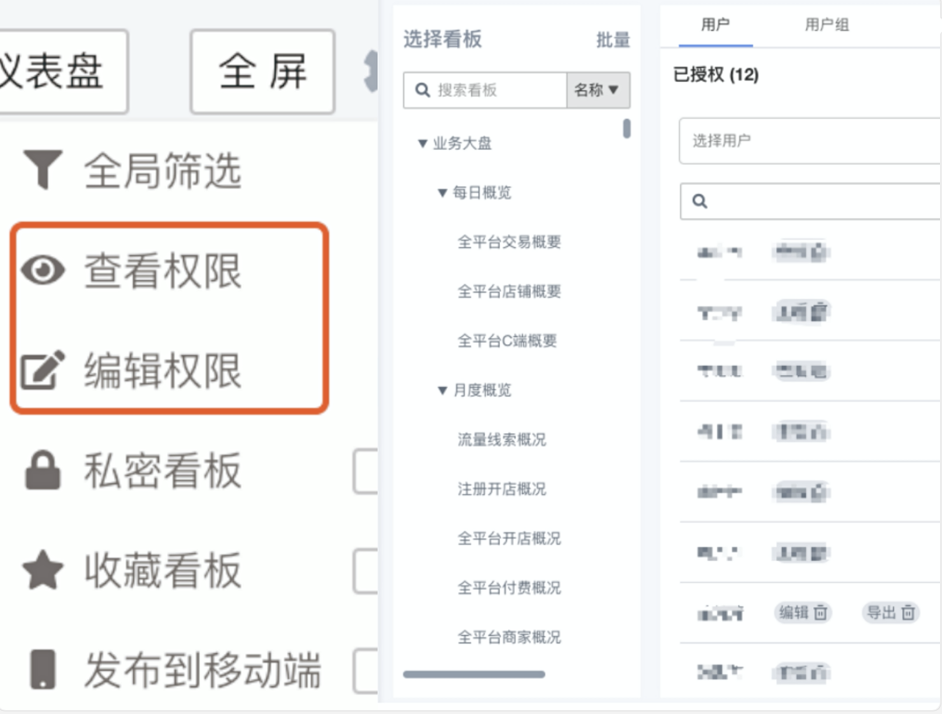## 2.3 图表类型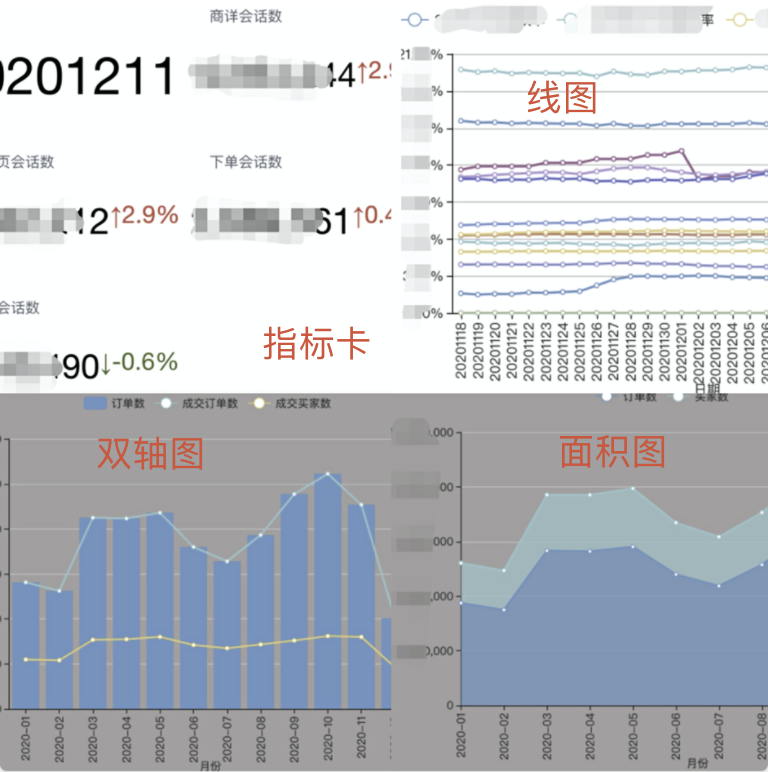• 指标卡可以用来描述指标的数值，也可以描述数据的变化趋势。
• 线图是对数据随时间变化的趋势描述，也可以描述多组数据随时间变化的趋势，如“近一年内某店铺的销售量和销售额趋势变化”。
• 双轴图是柱状图和线图的组合，适合对业务上关联较强的指标进行对比分析使用。
• 面积图也是对数据随时间变化的趋势描述，在“线图”的基础上，将线和自变量的轴之间的区域使用颜色填充，对趋势变化的描述更加突出。
• 堆积面积图和面积图的区别在于，每个指标数据量的起点是基于上一个指标数据量的，因此各个叠起来的面积表示各个指标数据量，堆积面积图整体代表了所有指标数据量的总和。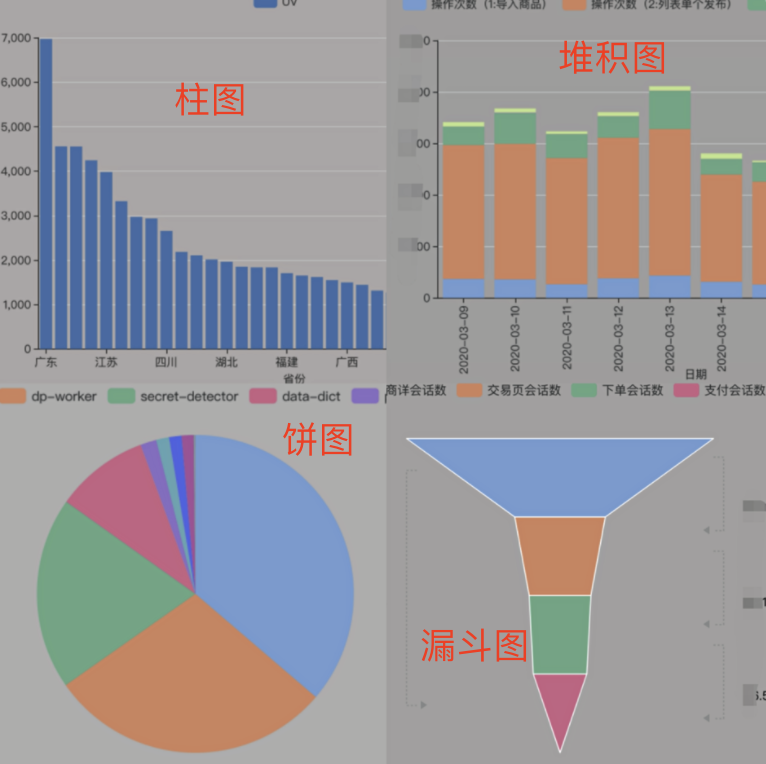• 柱状图是对分类数据的描述，又分为垂直柱状图和水平柱状图，分别用垂直或水平柱子来区分不同类别的数值。
• 堆积图是在分类数据的基础上，对每个分类再进行小分类的划分，是对大分类下小分类数据的描述，将每个柱子分割，分为垂直堆积图和水平堆积图，形象地表示了相同大分类下的各个小分类的数据分布情况。
• 饼图是对分类数据的占比情况描述，根据分类数据的占比将圆分为多个区块，通过区块弧度的大小来表示占比的多少，所有区块加和为100%。
• 漏斗图表示随着业务流程推进数据的流转情况，从上到下有逻辑上的顺序关系，用梯形面积表示各个环境的差异，梯形的上底和下底分别表示数据的流入和流出。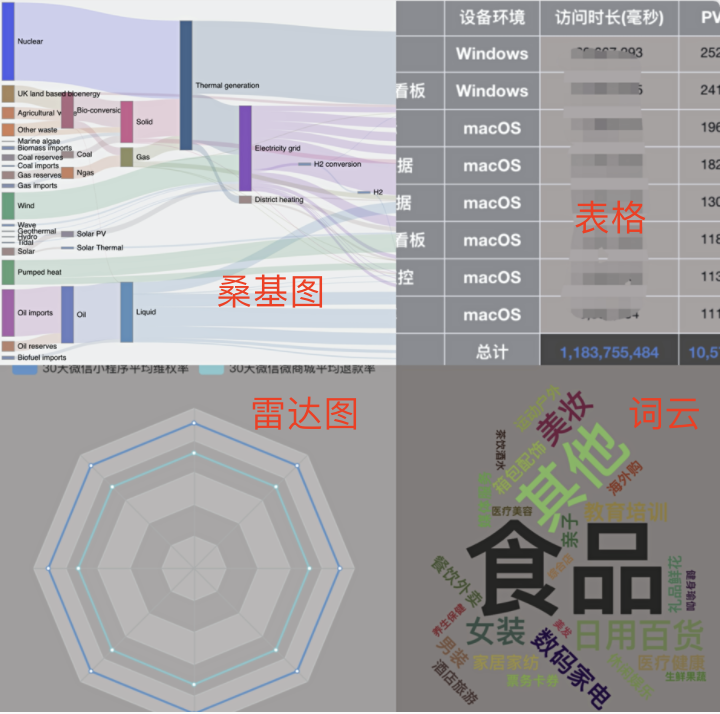图1.9 图表类型-3

• 桑基图又称能量分流图，表示一组值向另一组值的流向，可以方便地展现数据流动的方向。其中，边表示流动的数据，流量表示流动数值，节点表示不同的分类，边宽与流量成比例显示，由此可见，无论数据怎样流动，数据总值是不变的，遵循数据的“能量守恒”。

• 雷达图又称蜘蛛网图，是用于表现多维数据的图表，各维的数据映射到以圆心为起点，以圆周边缘为终点的坐标轴上，将同维的点用线连接，组成雷达图，雷达图所围面积是观察数据的关注点。
• 词云，又称文字云。将文本数据中词的重要性通过不同的颜色、大小等表示出来，使用户快速感知突出的数据，获取数据的价值。

## 2.4 筛选与排序

• 筛选：

• 可以对字段或数值进行筛选，包括对原生字段、计算字段、及字段聚合后的数据进行过滤，如筛选出“订单状态”为“交易成功”，交易额大于1000的订单信息。
• 目前支持的筛选条件有基本的>、>=、<、<=、=、<>、is null、is not null、between、like、not like、in等条件。同时可对时间字段按年、月、季度、周等不同的时间维度去观察数据，只需要对字段标记日期类型及相应的日期格式即可。
• 后续会支持条件表达式，使用户对条件的筛选更加灵活。目前支持如下日期类型及格式：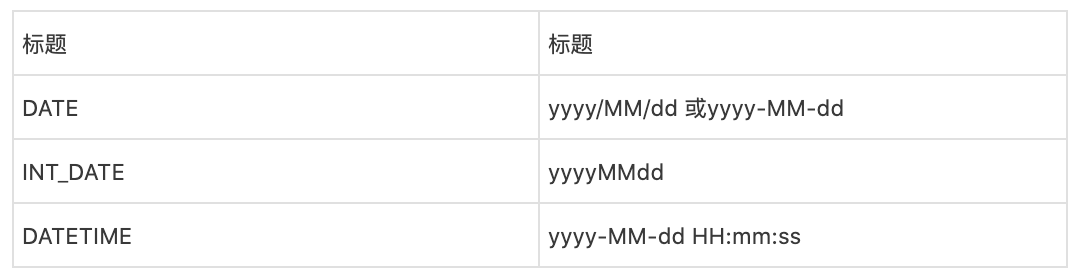图2.0 日期类型及格式

• 排序：

• 将指标按某种排列顺序进行排序，如按店铺的销售额降序排列出店铺的信息，这时可对指标进行排序。
• 目前支持对行维、指标的升降序排序。

## 2.7 行列权限

• 列权限：对不同的人或组设置不可见的字段，当访问的报表有用户不可见字段时，会友情提示用户申请字段权限。
• 行权限：对不同的人或组设置可见的数据，可通过“条件模式”，“自由模式”两种模式设置条件。如下图：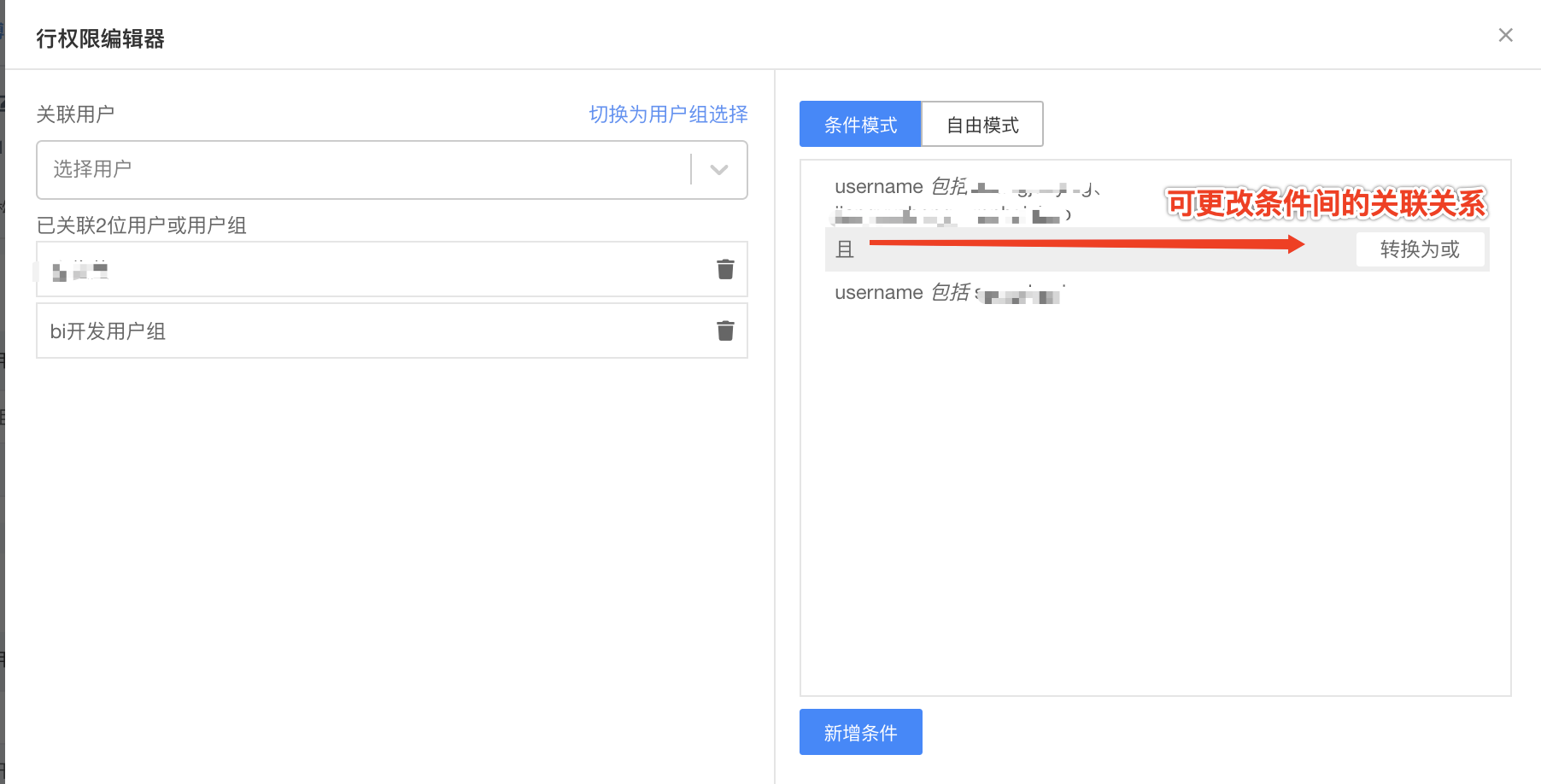图2.4 行列权限-条件模式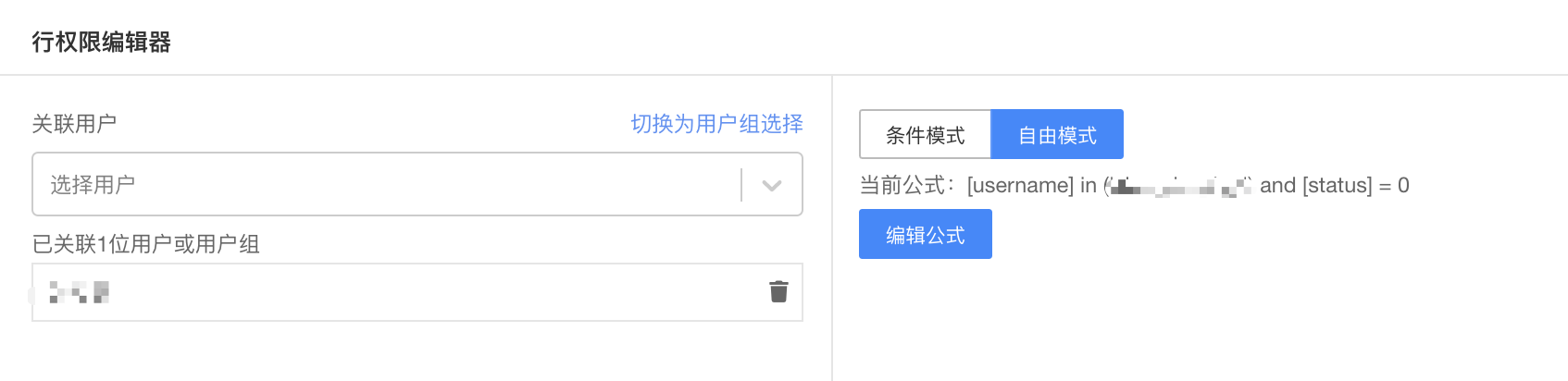图2.5 行列权限-自由模式

## 三、实现原理

### 3.1 行维度、数值、筛选、排序与分页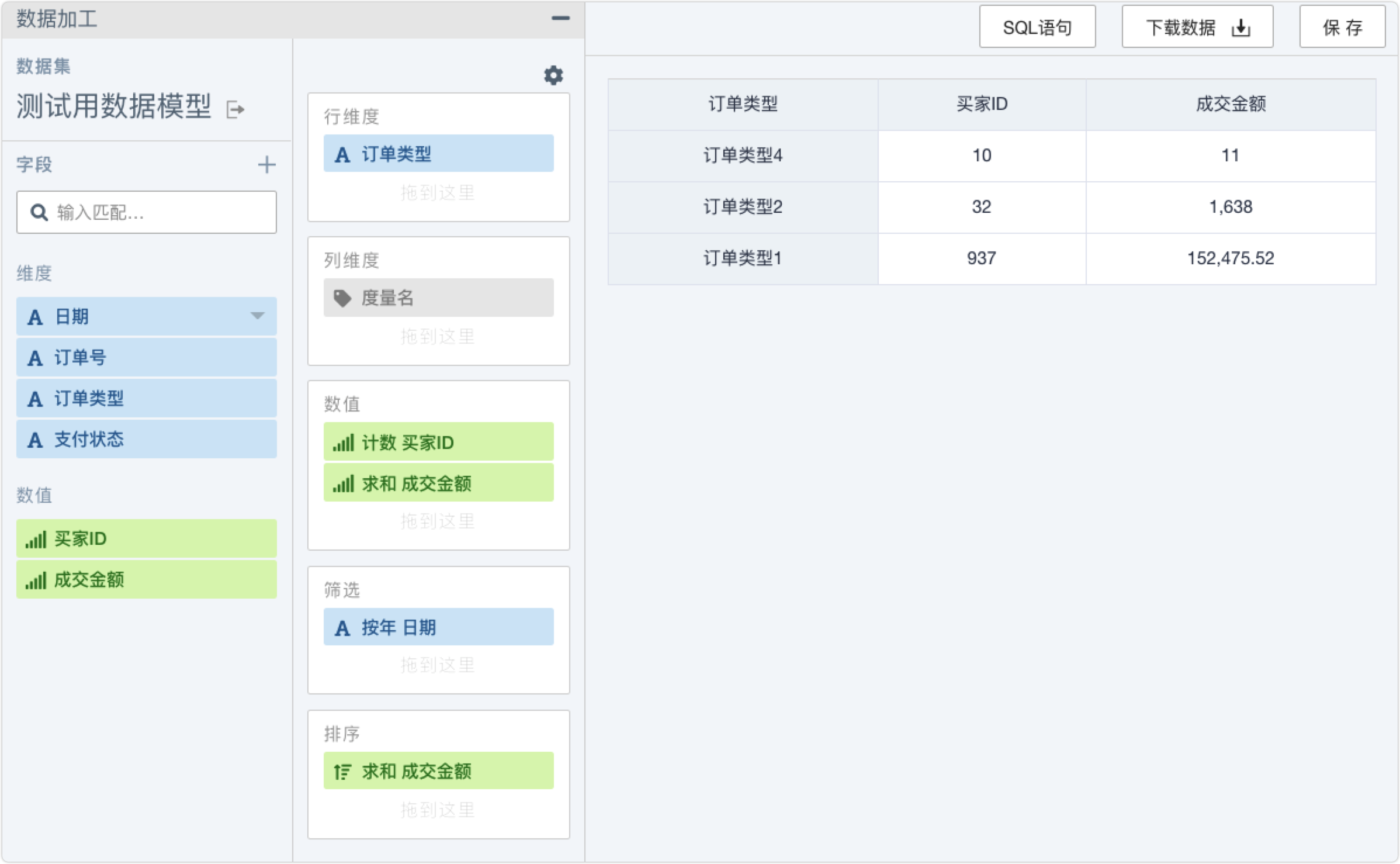SELECT *
FROM (
SELECT *, row_number() OVER () AS bi_rownum
FROM (
SELECT order_type AS bi_rowdim0, COUNT(buyer_id) AS bi_metric0, SUM(gmv) AS bi_metric1
FROM (
SELECT *
FROM dm_zbk.da_self_analysis_demo
) mid_dataset
WHERE the_date >= '2020-01-01'
AND the_date <= '2020-12-31'
GROUP BY order_type
ORDER BY bi_metric1 ASC
)
)
WHERE bi_rownum > 0
AND bi_rownum <= 10


### 3.2 列维度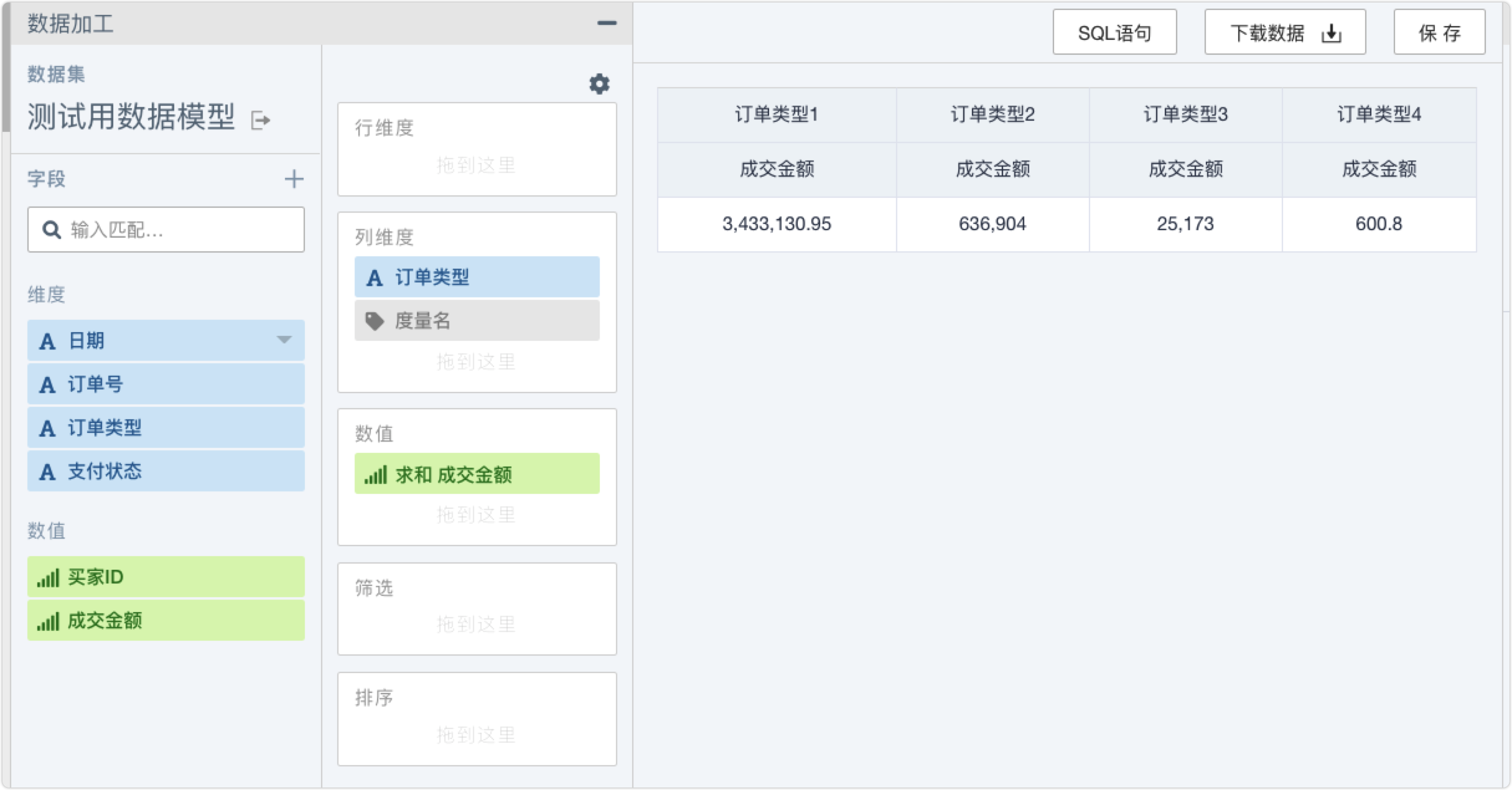SELECT SUM(if(order_type = '订单类型1', gmv, 0)) AS bi_metric0
, SUM(if(order_type = '订单类型2', gmv, 0)) AS bi_metric1
, SUM(if(order_type = '订单类型3', gmv, 0)) AS bi_metric2
, SUM(if(order_type = '订单类型4', gmv, 0)) AS bi_metric3
FROM (
SELECT *
FROM dm_zbk.da_self_analysis_demo
) mid_dataset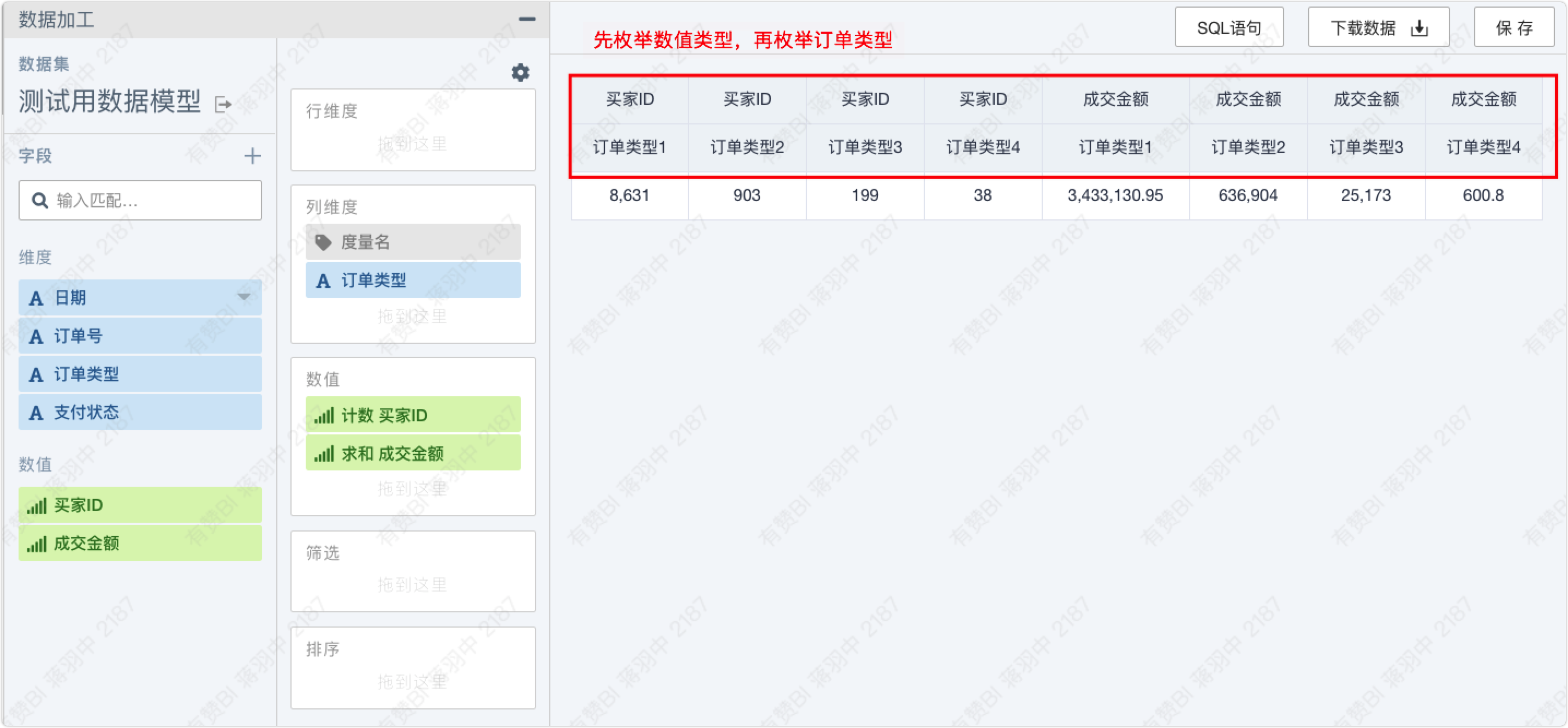“订单类型”在度量名上时的SQL如下：

        SELECT COUNT(if(order_type = '订单类型1', buyer_id, NULL)) AS bi_metric0
, SUM(if(order_type = '订单类型1', gmv, 0)) AS bi_metric1
, COUNT(if(order_type = '订单类型2', buyer_id, NULL)) AS bi_metric2
, SUM(if(order_type = '订单类型2', gmv, 0)) AS bi_metric3
, COUNT(if(order_type = '订单类型3', buyer_id, NULL)) AS bi_metric4
, SUM(if(order_type = '订单类型3', gmv, 0)) AS bi_metric5
, COUNT(if(order_type = '订单类型4', buyer_id, NULL)) AS bi_metric6
, SUM(if(order_type = '订单类型4', gmv, 0)) AS bi_metric7
FROM (
SELECT *
FROM dm_zbk.da_self_analysis_demo
) mid_dataset


        SELECT COUNT(if(order_type = '订单类型1', buyer_id, NULL)) AS bi_metric0
, COUNT(if(order_type = '订单类型2', buyer_id, NULL)) AS bi_metric1
, COUNT(if(order_type = '订单类型3', buyer_id, NULL)) AS bi_metric2
, COUNT(if(order_type = '订单类型4', buyer_id, NULL)) AS bi_metric3
, SUM(if(order_type = '订单类型1', gmv, 0)) AS bi_metric4
, SUM(if(order_type = '订单类型2', gmv, 0)) AS bi_metric5
, SUM(if(order_type = '订单类型3', gmv, 0)) AS bi_metric6
, SUM(if(order_type = '订单类型4', gmv, 0)) AS bi_metric7
FROM (
SELECT *
FROM dm_zbk.da_self_analysis_demo
) mid_dataset


### 3.3 计算字段

SELECT try( sum(if(order_type = '订单类型3', gmv , 0)) ) AS bi_metric0, try( sum(if(order_type = '订单类型4', gmv , 0)) ) AS bi_metric1, try( sum(if(order_type = '订单类型2', gmv , 0)) ) AS bi_metric2, try( sum(if(order_type = '订单类型1', gmv , 0)) ) AS bi_metric3
FROM (
SELECT *
FROM  dm_zbk.da_self_analysis_demo
) mid_dataset


# 四、总结与展望

• 支持更丰富的图表类型
• 完善如小计、环比、预警、数据大屏、数据智能分析等高级功能
• 降低用户的使用成本，提升用户的交互体验